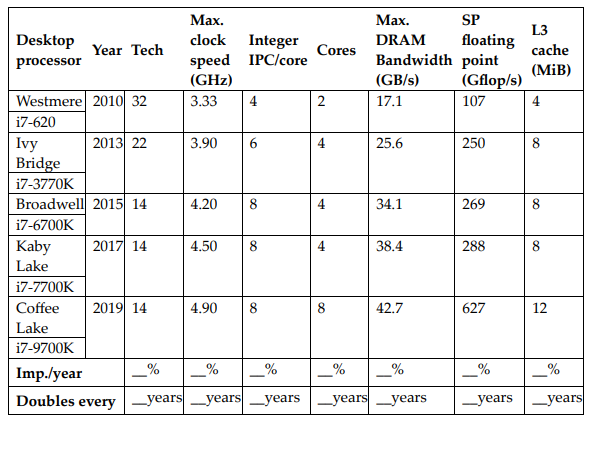# Question Solved1 AnswerConsider the table given next, which tracks several performance indicators for Intel desktop processors since 2010. The "Tech" column shows the minimum feature size of each processor's fabrication process. Assume that the die size has remained relatively constant and the number of transistors that comprise each processor scales at (1/t)^2, where t = theConsider the table given next, which tracks several performance indicators for Intel desktop processors since 2010. The "Tech" column shows the minimum feature size of each processor's fabrication process. Assume that the die size has remained relatively constant and the number of transistors that comprise each processor scales at (1/t)^2, where t = the minimum feature size. For each performance indicator, calculate the average rate of improvement from 2010 to 2019, as well as the number of years required to double each at that corresponding rate.

I already know the right answer because my prof. gave it to me.

For cores Imp./year is 15% and double years is 5 years.

However, I need to know how to get the the answer for the indicator cores.​​​​​​​

Transcribed Image Text: Consider the table given next, which tracks several performance indicators for Intel desktop processors since 2010. The "Tech" column shows the minimum feature size of each processor's fabrication process. Assume that the die size has remained relatively constant and the number of transistors that comprise each processor scales at (1/t)^2, where t = the
More
Transcribed Image Text: Consider the table given next, which tracks several performance indicators for Intel desktop processors since 2010. The "Tech" column shows the minimum feature size of each processor's fabrication process. Assume that the die size has remained relatively constant and the number of transistors that comprise each processor scales at (1/t)^2, where t = the